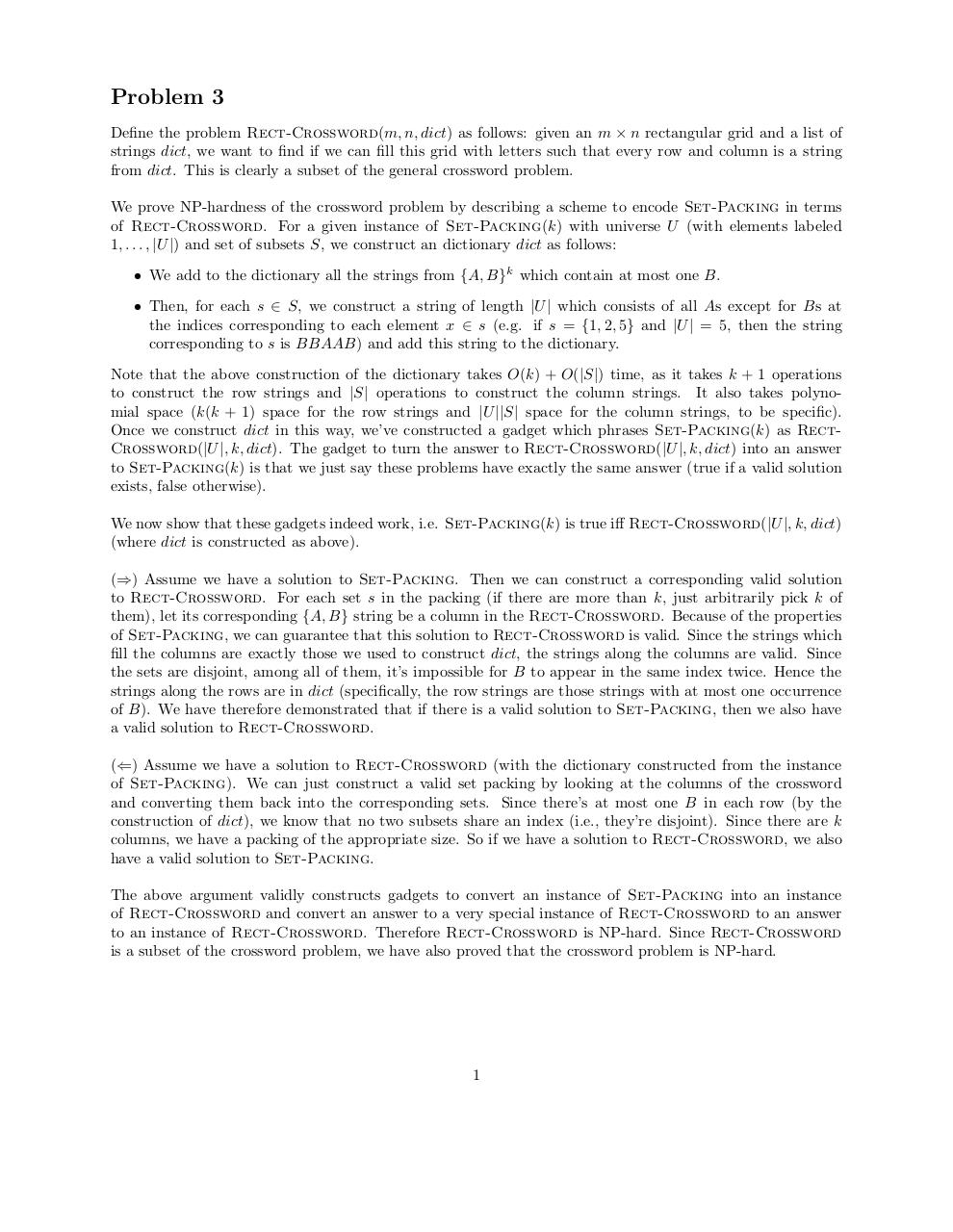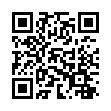# blah .pdf

### File information

Original filename: blah.pdf

This PDF 1.5 document has been generated by TeX / pdfTeX-1.40.15, and has been sent on pdf-archive.com on 28/03/2015 at 15:11, from IP address 76.24.x.x. The current document download page has been viewed 594 times.
File size: 68 KB (1 page).
Privacy: public file

blah.pdf (PDF, 68 KB)

### Document preview

Problem 3
Define the problem Rect-Crossword(m, n, dict) as follows: given an m × n rectangular grid and a list of
strings dict, we want to find if we can fill this grid with letters such that every row and column is a string
from dict. This is clearly a subset of the general crossword problem.
We prove NP-hardness of the crossword problem by describing a scheme to encode Set-Packing in terms
of Rect-Crossword. For a given instance of Set-Packing(k) with universe U (with elements labeled
1, . . . , |U |) and set of subsets S, we construct an dictionary dict as follows:
• We add to the dictionary all the strings from {A, B}k which contain at most one B.
• Then, for each s ∈ S, we construct a string of length |U | which consists of all As except for Bs at
the indices corresponding to each element x ∈ s (e.g. if s = {1, 2, 5} and |U | = 5, then the string
corresponding to s is BBAAB) and add this string to the dictionary.
Note that the above construction of the dictionary takes O(k) + O(|S|) time, as it takes k + 1 operations
to construct the row strings and |S| operations to construct the column strings. It also takes polynomial space (k(k + 1) space for the row strings and |U ||S| space for the column strings, to be specific).
Once we construct dict in this way, we’ve constructed a gadget which phrases Set-Packing(k) as RectCrossword(|U |, k, dict). The gadget to turn the answer to Rect-Crossword(|U |, k, dict) into an answer
to Set-Packing(k) is that we just say these problems have exactly the same answer (true if a valid solution
exists, false otherwise).
We now show that these gadgets indeed work, i.e. Set-Packing(k) is true iff Rect-Crossword(|U |, k, dict)
(where dict is constructed as above).
(⇒) Assume we have a solution to Set-Packing. Then we can construct a corresponding valid solution
to Rect-Crossword. For each set s in the packing (if there are more than k, just arbitrarily pick k of
them), let its corresponding {A, B} string be a column in the Rect-Crossword. Because of the properties
of Set-Packing, we can guarantee that this solution to Rect-Crossword is valid. Since the strings which
fill the columns are exactly those we used to construct dict, the strings along the columns are valid. Since
the sets are disjoint, among all of them, it’s impossible for B to appear in the same index twice. Hence the
strings along the rows are in dict (specifically, the row strings are those strings with at most one occurrence
of B). We have therefore demonstrated that if there is a valid solution to Set-Packing, then we also have
a valid solution to Rect-Crossword.
(⇐) Assume we have a solution to Rect-Crossword (with the dictionary constructed from the instance
of Set-Packing). We can just construct a valid set packing by looking at the columns of the crossword
and converting them back into the corresponding sets. Since there’s at most one B in each row (by the
construction of dict), we know that no two subsets share an index (i.e., they’re disjoint). Since there are k
columns, we have a packing of the appropriate size. So if we have a solution to Rect-Crossword, we also
have a valid solution to Set-Packing.
The above argument validly constructs gadgets to convert an instance of Set-Packing into an instance
of Rect-Crossword and convert an answer to a very special instance of Rect-Crossword to an answer
to an instance of Rect-Crossword. Therefore Rect-Crossword is NP-hard. Since Rect-Crossword
is a subset of the crossword problem, we have also proved that the crossword problem is NP-hard.

1Use the permanent link to the download page to share your document on Facebook, Twitter, LinkedIn, or directly with a contact by e-Mail, Messenger, Whatsapp, Line..

Use the short link to share your document on Twitter or by text message (SMS)

#### HTML Code

Copy the following HTML code to share your document on a Website or Blog

#### QR Code### Related keywords